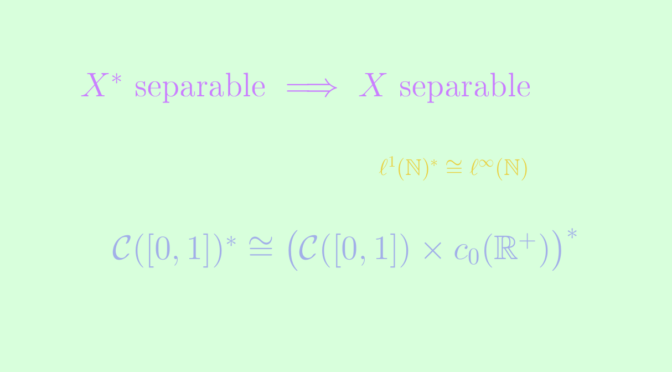# Separability of a vector space and its dual

Let’s recall that a topological space is separable when it contains a countable dense set. A link between separability and the dual space is following theorem:

Theorem: If the dual $$X^*$$ of a normed vector space $$X$$ is separable, then so is the space $$X$$ itself.

Proof outline: let $${f_n}$$ be a countable dense set in $$X^*$$ unit sphere $$S_*$$. For any $$n \in \mathbb{N}$$ one can find $$x_n$$ in $$X$$ unit ball such that $$f_n(x_n) \ge \frac{1}{2}$$. We claim that the countable set $$F = \mathrm{Span}_{\mathbb{Q}}(x_0,x_1,…)$$ is dense in $$X$$. If not, we would find $$x \in X \setminus \overline{F}$$ and according to Hahn-Banach theorem there would exist a linear functional $$f \in X^*$$ such that $$f_{\overline{F}} = 0$$ and $$\Vert f \Vert=1$$. But then for all $$n \in \mathbb{N}$$, $$\Vert f_n-f \Vert \ge \vert f_n(x_n)-f(x_n)\vert = \vert f_n(x_n) \vert \ge \frac{1}{2}$$. A contradiction since $${f_n}$$ is supposed to be dense in $$S_*$$.

We prove that the converse is not true, i.e. a dual space can be separable, while the space itself may be separable or not.

## Introducing some normed vector spaces

Given a closed interval $$K \subset \mathbb{R}$$ and a set $$A \subset \mathbb{R}$$, we define the $$4$$ following spaces. The first three are endowed with the supremum norm, the last one with the $$\ell^1$$ norm.

• $$\mathcal{C}(K,\mathbb{R})$$, the space of continuous functions from $$K$$ to $$\mathbb{R}$$, is separable as the polynomial functions with coefficients in $$\mathbb{Q}$$ are dense and countable.
• $$\ell^{\infty}(A, \mathbb{R})$$ is the space of real bounded functions defined on $$A$$ with countable support.
• $$c_0(A, \mathbb{R}) \subset \ell^{\infty}(A, \mathbb{R})$$ is the subspace of elements of $$\ell^{\infty}(A)$$ going to $$0$$ at $$\infty$$.
• $$\ell^1(A, \mathbb{R})$$ is the space of summable functions on $$A$$: $$u \in \mathbb{R}^{A}$$ is in $$\ell^1(A, \mathbb{R})$$ iff $$\sum \limits_{a \in A} |u_x| < +\infty$$.

When $$A = \mathbb{N}$$, we find the usual sequence spaces. It should be noted that $$c_0(A, \mathbb{R})$$ and $$\ell^1(A, \mathbb{R})$$ are separable iff $$A$$ is countable (otherwise the subset $$\big\{x \mapsto 1_{\{a\}}(x),\ a \in A \big\}$$ is uncountable, and discrete), and that $$\ell^{\infty}(A, \mathbb{R})$$ is separable iff $$A$$ is finite (otherwise the subset $$\{0,1\}^A$$ is uncountable, and discrete).

We conclude this introduction by reminding that the dual space of $$c_0(A, \mathbb{R})$$ is $$\ell^1(A, \mathbb{R})$$, and the dual of $$\ell^1(A, \mathbb{R})$$ is $$\ell^{\infty}(A, \mathbb{R})$$.

## Separable space with non separable dual

An immediate counterexample to the reciprocal of above theorem is the space $$\ell^1(\mathbb{N}, \mathbb{R})$$. It is separable while its dual $$\ell^{\infty}(\mathbb{N}, \mathbb{R})$$ is not.

## Separable and non separable space with same dual

A further counterexample is that a separable and a non separable space can have isometrically isomorphic dual spaces. This example comes from Bill Johnson post on MathOverflow.

Consider $$E := \mathcal{C}([0,1],\mathbb{R})$$ endowed with the supremum norm. According to Riesz_representation_theorem the dual of $$E$$ is the space $$\mathcal{M}([0,1])$$ of regular signed bounded countably additive measures on $$[0,1]$$ endowed with the total variation norm.
Any continuous functional $$L \in E^*$$ can be represented as $$L(f) = \displaystyle{\int_0^1} f(t)\mu(dt)$$ for some signed measure $$\mu \in \mathcal{M}([0,1])$$.

The second of our two spaces is $$F := \mathcal{C}([0,1],\mathbb{R}) \times c_0(\mathbb{R}^+, \mathbb{R})$$ endowed with the maximum of the two supremum norms. Starting from now, all product spaces will implicitly be endowed with the sum of norms. The dual space of $$F$$ is $$\mathcal{M}([0,1]) \times \ell^1(\mathbb{R}^+,\mathbb{R})$$.

We will denote respectively $$\mathcal{M}^c([0,1])$$ and $$\mathcal{M}^d([0,1])$$ the subspaces of continuous measures (measure of any singleton is zero) and discrete measures (concentrated on a discrete set). Then the application \begin{aligned}[t] \mathcal{M}^c([0,1]) \times \mathcal{M}^d([0,1]) &\longrightarrow \mathcal{M}([0,1]) \\ (\mu_c, \mu_d) &\longmapsto \mu_c + \mu_d \end{aligned} is an isomorphism according to Lebesgue’s decomposition theorem. It is quite straightforward to show that $$||\mu_d + \mu_c||_{TV} = ||\mu_d||_{TV} + ||\mu_c||_{TV}$$, so the isomorphism is an isometry. Hence $$F^*$$ is isometrically isomorphic to:

• $$\mathcal{M}^c([0,1]) \times \mathcal{M}^d([0,1]) \times \ell^1(\mathbb{R}^+,\mathbb{R})$$,
• $$\mathcal{M}^c([0,1]) \times \mathcal{M}^d([0,1]) \times \ell^1(]1,2],\mathbb{R})$$ as $$]1,2]$$ is homeomorph to $$\mathbb{R}^+$$,
• $$\mathcal{M}^c([0,1]) \times \mathcal{M}^d([0,1]) \times \mathcal{M}^d(]1,2])$$ thanks to the isometry $$f \in \ell^1(]1,2],\mathbb{R}) \mapsto \sum \limits_{x \in ]1,2]} f(x) \delta_x$$,
• $$\mathcal{M}^c([0,1]) \times \mathcal{M}^d([0,2])$$ by concatenating the discrete measures,
• $$\mathcal{M}^c([0,1]) \times \mathcal{M}^d([0,1])$$, as $$[0,1]$$ is homeomorph to $$[0,2]$$.

We conclude that the dual of $$F$$ is isometrically isomorphic to $$\mathcal{M}([0,1])$$, the dual of $$E$$. Note finally that $$E$$ is separable, while $$F$$ is not since it contains $$\{0\} \times c_0(\mathbb{R}^+, \mathbb{R})$$ which is not separable.